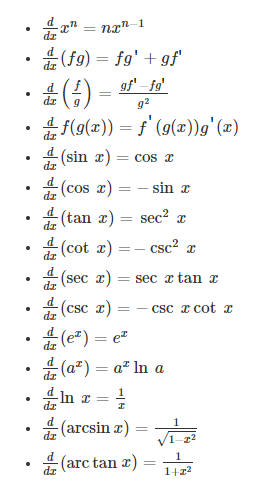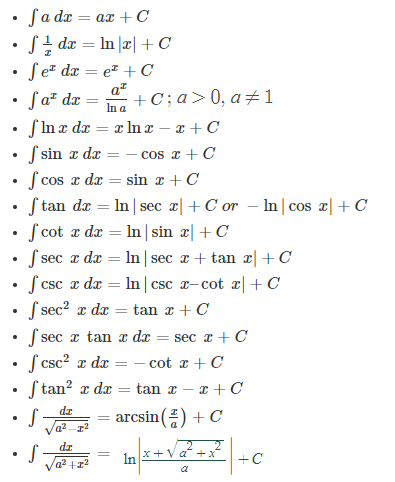# Calculus Formulas

Calculus is one of the branches of Mathematics that is involved in the study of ‘Rate of Change’ and their application to solving equations. It has two major branches, Differential Calculus that is concerning rates of change and slopes of curves, and Integral Calculus concerning accumulation of quantities and the areas under and between curves.

Both branches make use of the fundamental notions of convergence of infinite sequences and infinite series to a well-defined limit. These two branches are related to each other by the fundamental theorem of calculus.

The Differential Calculus splits up an area into small parts to calculate the rate of change. The Integral calculus joins small parts to calculates the area or volume and in short, is the method of reasoning or calculation. In this page, you can see a list of Calculus Formulas such as integral formula, derivative formula, limits formula etc.

Since calculus plays an important role to get the optimal solution, it involves lots of calculus formulas concerned with the study of the rate of change of quantities. It describes the rate of change of a function for the given input value using the derivative of a function. The process of finding the derivative of a function is called differentiation. The reverse process of the differentiation is called integration. This process helps to maximize or minimize the function for some set, it often represents the different range of choices for some specific conditions. The function allows us to compare the different choices where it uses different calculus formulas to chooses the best optimal solution.

## Differential Calculus Formulas

Differentiation is a process of finding the derivative of a function. The derivative of a function is defined as y = f(x) of a variable x, which is the measure of the rate of change of a variable y changes with respect to the change of variable x. It means that the derivative of a function with respect to the variable x. There are a number of rules to find the derivative of a function. These rules make the differentiation process easier for different functions such as trigonometric functions, logarithmic functions, etc. Here, a list of differential calculus formulas is given below:## Integral Calculus Formulas

The basic use of integration is to add the slices and make it into a whole thing. In other words, integration is the process of continuous addition and the variable “C” represents the constant of integration. But often, integration formulas are used to find the central points, areas and volumes for the most important things. Also, it helps to find the area under the curve of a function. There are certain important integral calculus formulas helps to get the solutions. These integral calculus formulas help to minimize the time taken to solve the problem. The list of integral calculus formulas is given below:To learn more formulas on differential and integral calculus, register with BYJU’S – The Learning App and download the app to learn with ease.